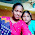SA 2 Mathematics Key by SCERT SA-2 Maths Principles of Evaluation

SA 2 Mathematics Key by SCERT. SCERT has released the Maths Key for Maths Summative-2 Exam. Class Wise Key is given below. SA 2 Mathematics Key by SCERT SA-2 Maths Principles of Evaluation. SA-2 6th Exam Key, SA-2 7th Maths Exam Key, SA-2 8th Maths Exam Key, 9th Class SA-2 Mathematics Exam Key.

SA 2 Mathematics Key SA-2 Maths Principles of Evaluation

1.This comment has been removed by the author.

2.In AP SA-II X Class mathematics paper - II key , we observe three mistakes in part-B
i.e.(22. B, 27.A, 30.D)

In AP SA-II,. IX class mathematics paper-I key, we observe one mistake in part-B, i.e. 29.A

R Sreenivasa Rao, SA(Maths), ZPHS, Vissannapeta